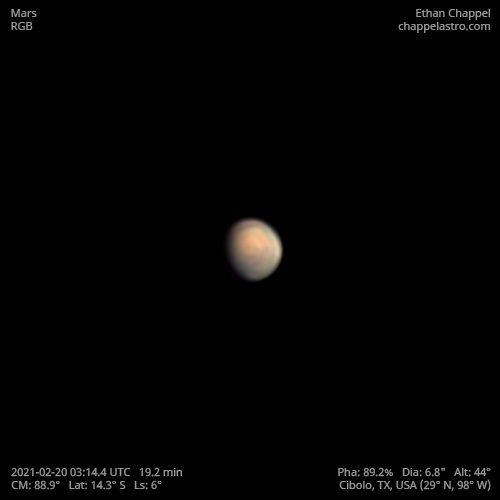# Mars 2021-02-20 03:14 UTC

CM1: 88.90°

CLat: -14.30°

Description

Imaging Mars after a tough week was a nice change of pace. Historic snow storms brought an unusually long and intense cold snap to Texas, causing much of the state to fall into rolling blackouts for days. We lost home internet access for 5 days and the cell signal became too weak for a reliable connection. Snow was brought inside to melt in case the local water supply ran dry. The air outside was a little colder than normal and small patches of snow were on the ground tonight. Seeing was good enough to get an acceptable image with enough data.

Mars passed vernal equinox two weeks ago, bringing spring to the northern hemisphere and autumn to the south. The planet is now 200 million kilometers away and just over a fourth of the angular diameter it was at opposition in October. Valles Marineris can be seen near the center of the disk.

These images were taken less than two days after Perseverance touched down on the red planet. The landing site was facing away from us when taking these images.

Equipment

ZWO ASI290MM

Celestron EdgeHD 8

Logs
```FireCapture v2.7 02 BETA Settings
------------------------------------
Observer=Ethan Chappel
Location=Cibolo
Scope=Celestron C8 EdgeHD
Camera=ZWO ASI290MM
Filter=G
Profile=Mars
Diameter=6.78"
Magnitude=0.78
CM=89.2°  (during mid of capture)
FocalLength=4650mm (F/13)
Resolution=0.13"
Filename=m2021-02-20_03-14-27_g_ec.ser
Date=2021_02_20
Start=03_11_57.695
Mid=03_14_27.697
End=03_16_57.699
Start(UT)=03_11_57.695
Mid(UT)=03_14_27.697
End(UT)=03_16_57.699
Duration=300.004s
Date_format=yyyy_MM_dd
Time_format=HH_mm_ss
LT=UT
Frames captured=37078
File type=SER
Binning=1x1
ROI=160x160
ROI(Offset)=768x344
FPS (avg.)=123
Shutter=8.000ms
Gain=290 (48%)
AutoExposure=off
AutoGain=off
AutoHisto=75 (off)
Brightness=1
FPS=100 (off)
Gamma=50 (off)
HighSpeed=on
SoftwareGain=10 (off)
USBTraffic=100
Histogramm(min)=0
Histogramm(max)=179
Histogramm=70%
Noise(avg.deviation)=0.73
Limit=300 Seconds
Sensor temperature=57.2°F
Focuser position=28470
FireCapture v2.7 02 BETA Settings
------------------------------------
Observer=Ethan Chappel
Location=Cibolo
Scope=Celestron C8 EdgeHD
Camera=ZWO ASI290MM
Filter=R
Profile=Mars
Diameter=6.78"
Magnitude=0.78
CM=87.5°  (during mid of capture)
FocalLength=5100mm (F/14)
Resolution=0.12"
Filename=m2021-02-20_03-07-20_r_ec.ser
Date=2021_02_20
Start=03_04_50.279
Mid=03_07_20.283
End=03_09_50.288
Start(UT)=03_04_50.279
Mid(UT)=03_07_20.283
End(UT)=03_09_50.288
Duration=300.009s
Date_format=yyyy_MM_dd
Time_format=HH_mm_ss
LT=UT
Frames captured=49978
File type=SER
Binning=1x1
ROI=160x160
ROI(Offset)=768x344
FPS (avg.)=166
Shutter=6.000ms
Gain=280 (46%)
AutoExposure=off
AutoGain=off
AutoHisto=75 (off)
Brightness=1
FPS=100 (off)
Gamma=50 (off)
HighSpeed=on
SoftwareGain=10 (off)
USBTraffic=100
Histogramm(min)=0
Histogramm(max)=225
Histogramm=88%
Noise(avg.deviation)=0.70
Limit=300 Seconds
Sensor temperature=58.6°F
Focuser position=28480
FireCapture v2.7 02 BETA Settings
------------------------------------
Observer=Ethan Chappel
Location=Cibolo
Scope=Celestron C8 EdgeHD
Camera=ZWO ASI290MM
Filter=B
Profile=Mars
Diameter=6.78"
Magnitude=0.78
CM=91.4°  (during mid of capture)
FocalLength=4950mm (F/13)
Resolution=0.12"
Filename=m2021-02-20_03-23-29_b_ec.ser
Date=2021_02_20
Start=03_22_59.468
Mid=03_23_29.629
End=03_23_59.790
Start(UT)=03_22_59.468
Mid(UT)=03_23_29.629
End(UT)=03_23_59.790
Duration=60.322s
Date_format=yyyy_MM_dd
Time_format=HH_mm_ss
LT=UT
Frames captured=10046
File type=SER
Binning=1x1
ROI=160x160
ROI(Offset)=768x344
FPS (avg.)=166
Shutter=6.000ms
Gain=380 (63%)
AutoExposure=off
AutoGain=off
AutoHisto=75 (off)
Brightness=1
FPS=100 (off)
Gamma=50 (off)
HighSpeed=on
SoftwareGain=10 (off)
USBTraffic=100
Histogramm(min)=0
Histogramm(max)=191
Histogramm=74%
Noise(avg.deviation)=1.73
Limit=240 Seconds
Sensor temperature=55.6°F
Focuser position=28510
FireCapture v2.7 02 BETA Settings
------------------------------------
Observer=Ethan Chappel
Location=Cibolo
Scope=Celestron C8 EdgeHD
Camera=ZWO ASI290MM
Filter=B
Profile=Mars
Diameter=6.78"
Magnitude=0.78
CM=90.8°  (during mid of capture)
FocalLength=4950mm (F/13)
Resolution=0.12"
Filename=m2021-02-20_03-20-55_b_ec.ser
Date=2021_02_20
Start=03_18_55.670
Mid=03_20_55.666
End=03_22_55.663
Start(UT)=03_18_55.670
Mid(UT)=03_20_55.666
End(UT)=03_22_55.663
Duration=239.993s
Date_format=yyyy_MM_dd
Time_format=HH_mm_ss
LT=UT
Frames captured=39977
File type=SER
Binning=1x1
ROI=160x160
ROI(Offset)=768x344
FPS (avg.)=166
Shutter=6.000ms
Gain=380 (63%)
AutoExposure=off
AutoGain=off
AutoHisto=75 (off)
Brightness=1
FPS=100 (off)
Gamma=50 (off)
HighSpeed=on
SoftwareGain=10 (off)
USBTraffic=100
Histogramm(min)=0
Histogramm(max)=183
Histogramm=71%
Noise(avg.deviation)=1.75
Limit=240 Seconds
Sensor temperature=55.9°F
Focuser position=28510
```